null 《Predict Anchor Links across Social Networks via an Embedding Approach》阅读笔记 - K码农

# 3|0Predict Anchor Links via Embedding

$G=\left\{V,E\right\}$$G=\lbrace V,E \rbrace$ 表示一个社交网络，$V$$V$ 表示节点集合，$E\subset \left(V×V\right)$$E\subset (V\times V)$ 表示边集合。在生活中，存在一些用户同时涉及到两个不同的社交网络中，从而两个社交网络间的锚链接。为了不失一般性，将其中一个网络作为源网络，另一个网络作为目标网络，分别用 ${G}^{s}$$G^s$${G}^{t}$$G^t$ 表示。对于源网络的每一个节点，我们的目的是在目标网络中识别其对应的节点（如果存在的话）。这可以正式表述为以下锚链接预测问题。

Anchor link prediction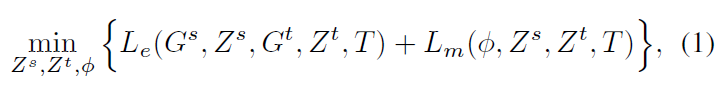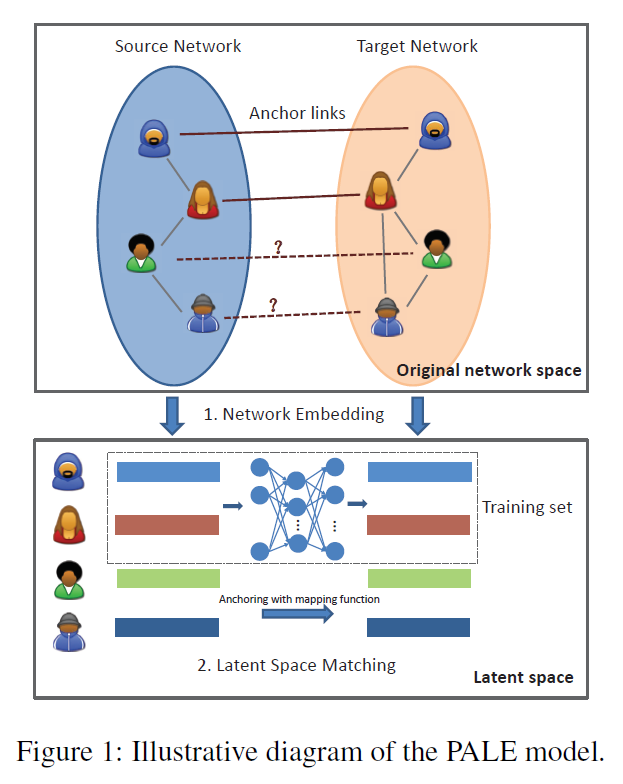## 3|1嵌入阶段

### 跨网络扩展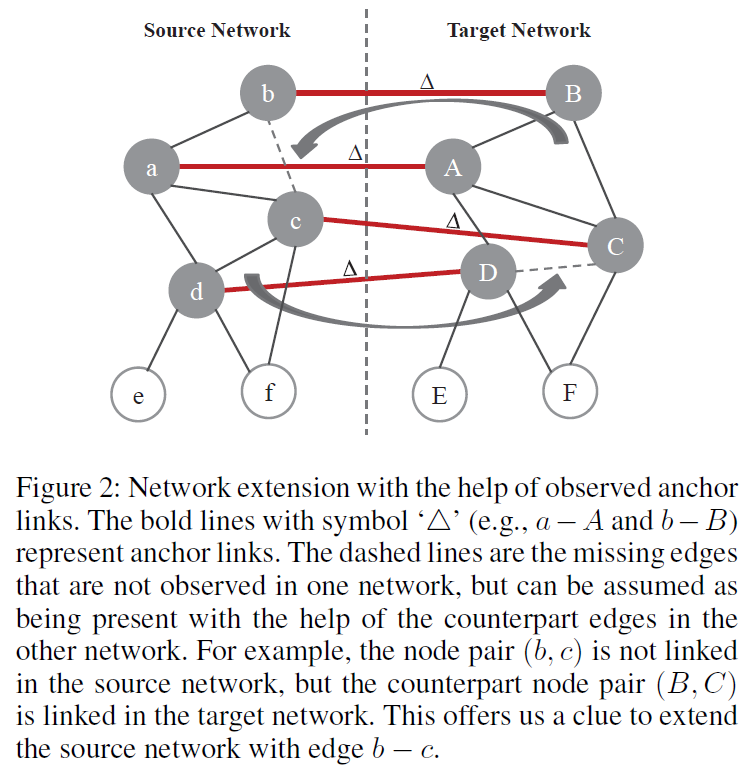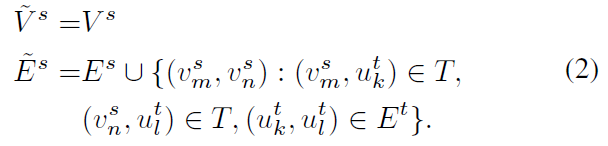### 网络嵌入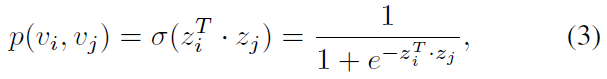$L=\prod _{\left(i,j\right)\in \stackrel{~}{E}}p\left({v}_{i},{v}_{j}\right)$

$\sum _{\left(i,j\right)\in \stackrel{~}{E}}\mathrm{log}p\left({v}_{i},{v}_{j}\right)=\sum _{\left(i,j\right)\in \stackrel{~}{E}}\mathrm{log}\sigma \left({z}_{i}^{T}\cdot {z}_{j}\right)$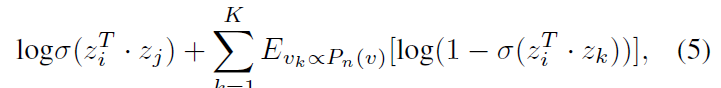### 负采样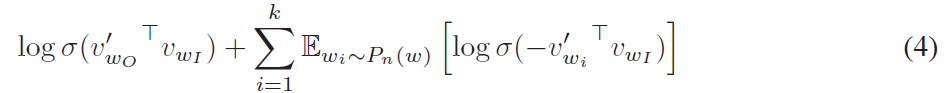## 3|2匹配阶段

${L}_{m}\left(\varphi ,{Z}^{s},{Z}^{t},T\right)=\sum _{\left({v}_{l}^{s},{u}_{n}^{t}\right)\in T}||\varphi \left({z}_{l}^{s};\mathrm{\Theta }\right)-{z}_{n}^{t}|{|}_{F}$

$\varphi \left({z}_{l}^{s};\mathrm{\Theta }\right)=\mathrm{\Theta }×{z}_{l}^{s}$

## 3|3锚链接预测

$\underset{n}{min}||\varphi \left({z}_{l}^{s};\mathrm{\Theta }\right)-{z}_{n}^{t}|{|}_{F}$

# 4|0实验

• Degree-Based Alignment：即依据节点的度来进行匹配，作为一个基线方法；
• Matching Across Domains（MAD）：它通过奇异值分解来匹配同构网络上的共享节点，采用无监督方法；
• Multi-Network Anchoring（MNA）：它从部分对齐的社交网络中提取成对的社交特征，然后将锚链接预测问题解决为分类问题；
• Collective Random Walk（CRW）：在具有锚链接的网络上进行随机游走以识别另一网络中的每个节点的对应物；
• PALE（LIN）：PALE 模型加上线性映射函数；
• PALE（MLP）：PALE 模型加上 MLP 作为非线性映射函数。

## 4|1基于 Facebook 的实验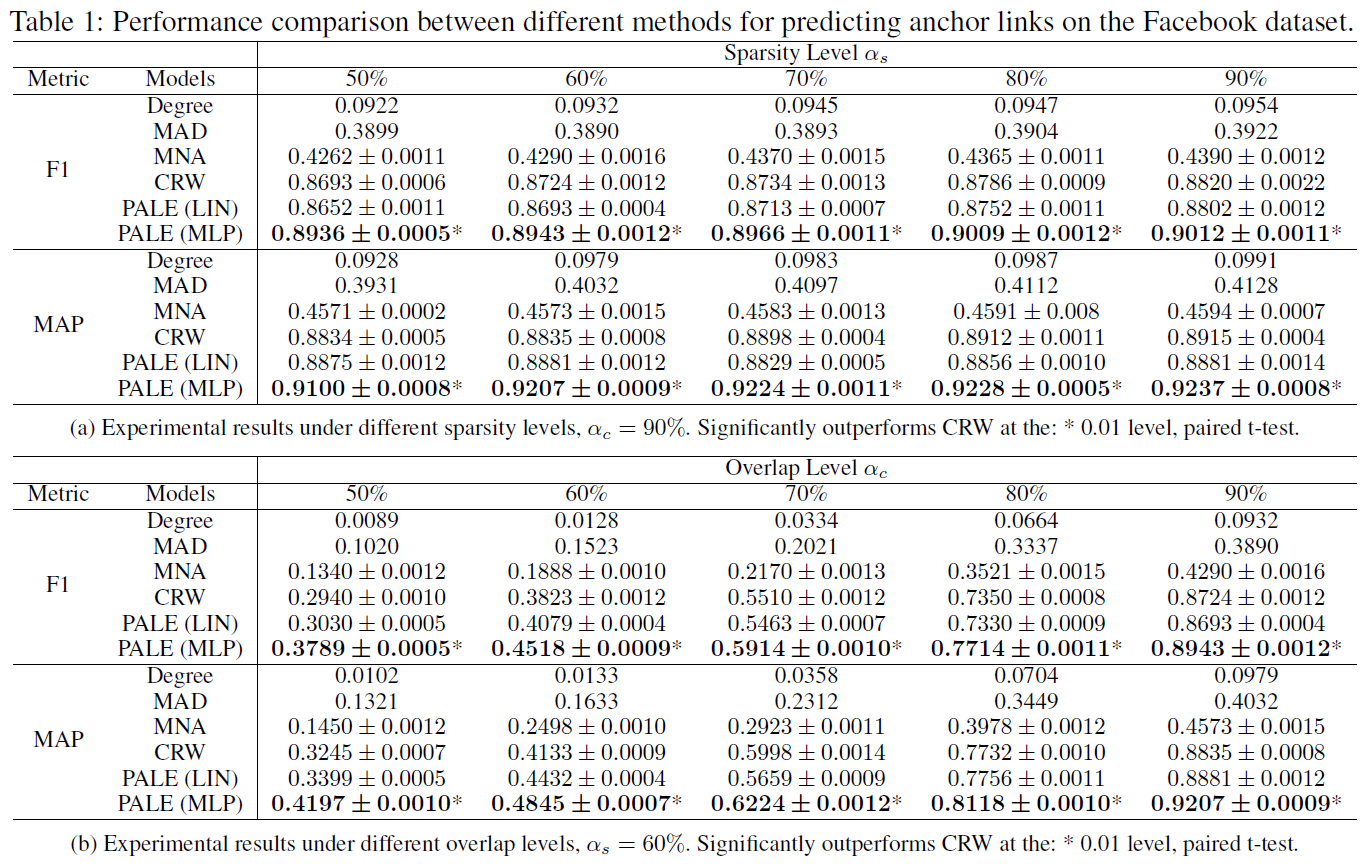## 4|2基于共同作者网络的实验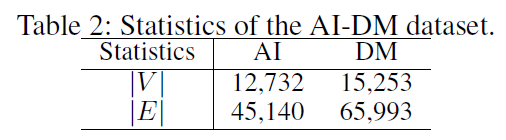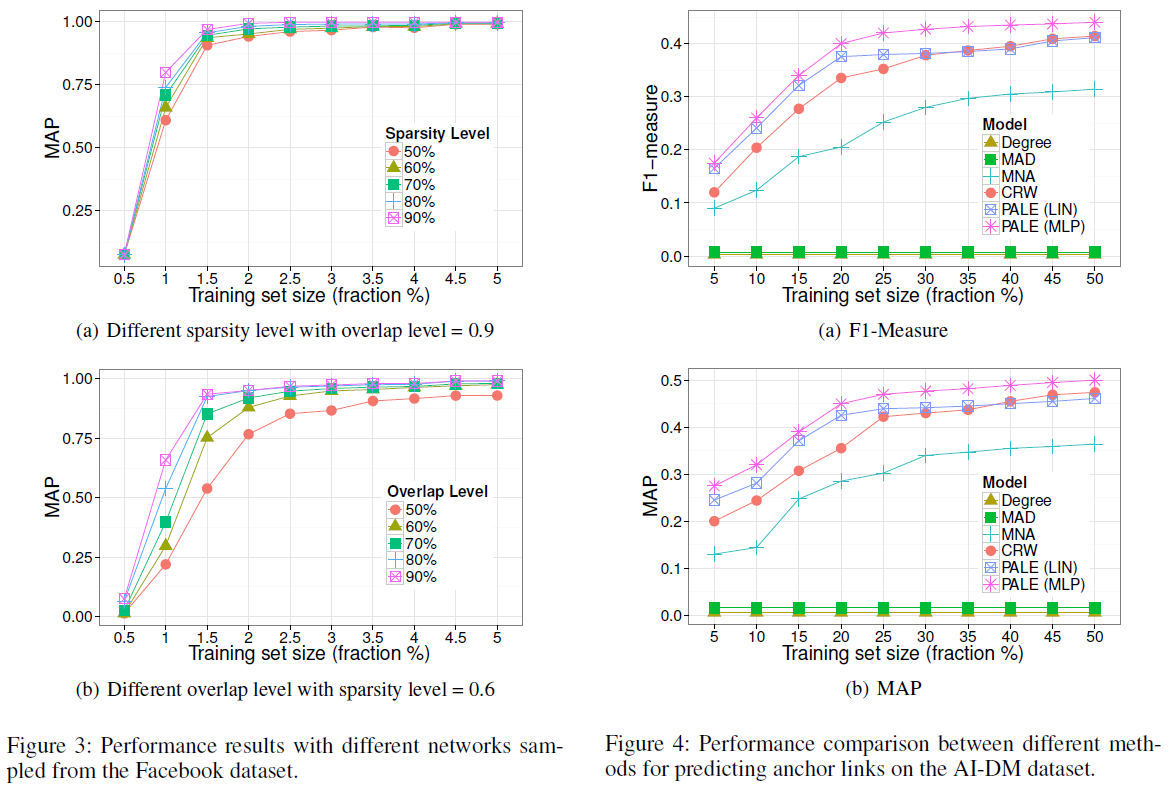__EOF__